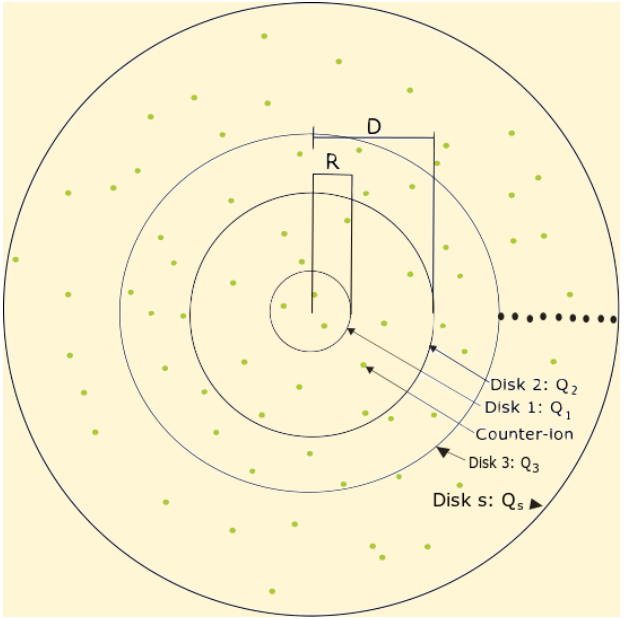## Two component plasma at Γ=2 : a random matrix theory semi-application

In this project we study some aspects of equilibrium statistical mechanics for a classical two-dimensional one-component Coulomb plasma (logarithmic interaction) with circular symmetry and N point-like charges. The model presented is an extension of the one studied by Mallarino and Téllez. The configuration is such that there are N particles of charge q each, constrained to lie all over a two dimensional plane, which has regions delimited by two charged disks such that Nq-Q1 – Q2 = 0. In this model, M counterions are inside the area demarcated by the first disk, additionally there are L ions between the two disks and finally P outside the second one. We focus our research in finding the correlation functions, the integrated charge and the contact density for a Coupling constant Γ=2, where the system is exactly solvable according to analogies between 2D Coulomb gases and Random Matrix Theory.

`Universidad de los Andes | Vigilada MineducaciónReconocimiento como Universidad: Decreto 1297 del 30 de mayo de 1964.Reconocimiento personería jurídica: Resolución 28 del 23 de febrero de 1949 Minjusticia.`